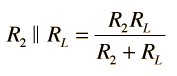# Voltage Divider

The two resistor voltage divider is used often to supply a voltage different from that of an available battery or power supply. In application the output voltage depends upon the resistance of the load it drives.whereis the parallel resistance of R2 and the load resistor RL.

 For R1 = Ω, R2 =Ω, and V1 = V,
 the open circuit output voltage would be Vout = V
 If the voltage divider is suppying a load RL = Ω,
 then the output circuit voltage is Vout = V

For this circuit, the total power supplied by the power supply is
Ptotal = watts

and the power delivered to the load resistor RL is
Pout = watts.